HomeCompositio Mathematica

# Subconvexity and equidistribution of Heegner points in the level aspect

Published online by Cambridge University Press:  17 June 2013

Corresponding

## Abstract

Let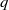$q$ be a prime and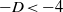$- D\lt - 4$ be an odd fundamental discriminant such that$q$ splits in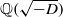$\mathbb{Q} ( \sqrt{- D} )$ . For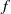$f$ a weight-zero Hecke–Maass newform of level$q$ and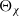${\Theta }_{\chi }$ the weight-one theta series of level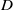$D$ corresponding to an ideal class group character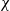$\chi$ of$\mathbb{Q} ( \sqrt{- D} )$ , we establish a hybrid subconvexity bound for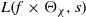$L(f\times {\Theta }_{\chi } , s)$ at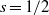$s= 1/ 2$ when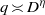$q\asymp {D}^{\eta }$ for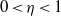$0\lt \eta \lt 1$ . With this circle of ideas, we show that the Heegner points of level$q$ and discriminant$D$ become equidistributed, in a natural sense, as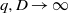$q, D\rightarrow \infty$ for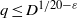$q\leq {D}^{1/ 20- \varepsilon }$ . Our approach to these problems is connected to estimating the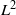${L}^{2}$ -restriction norm of a Maass form of large level$q$ when restricted to the collection of Heegner points. We furthermore establish bounds for quadratic twists of Hecke–Maass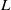$L$ -functions with simultaneously large level and large quadratic twist, and hybrid bounds for quadratic Dirichlet$L$ -functions in certain ranges.

## MSC classification

Type
Research Article
Information
Compositio Mathematica , July 2013 , pp. 1150 - 1174

## References

Biró, A., Cycle integrals of Maass forms of weight 0 and Fourier coefficients of Maass forms of weight 1/2, Acta Arith. 94 (2000), 103152; 54 (2004), 831–847.Google Scholar
Blomer, V., On the 4-norm of an automorphic form, J. Eur. Math. Soc. (JEMS), to appear, math.NT/1110.4717.Google Scholar
Blomer, V. and Harcos, G., Hybrid bounds for twisted$L$-functions, J. Reine Angew. Math. 621 (2008), 5379.Google Scholar
Blomer, V., Khan, R. and Young, M., Distribution of Mass of holomorphic cusp forms, Duke Math. J., to appear, Preprint (2012), math.NT/1203.2573.Google Scholar
Conrey, B. and Iwaniec, H., The cubic moment of central values of automorphic$L$-functions, Ann. of Math. (2) 151 (2000), 11751216.CrossRefGoogle Scholar
Duke, W., Hyperbolic distribution problems and half-integral weight Maass forms, Invent. Math. 92 (1988), 7390.CrossRefGoogle Scholar
Duke, W., Friedlander, J. and Iwaniec, H., Class group$L$-functions, Duke Math. J. 79 (1995), 156.CrossRefGoogle Scholar
Feigon, B. and Whitehouse, D., Averages of central$L$-values of Hilbert modular forms with an application to subconvexity, Duke Math. J. 149 (2009), 347410.CrossRefGoogle Scholar
Gelbart, S. and Jacquet, H., A relation between automorphic representations of$GL(2)$ and$GL(3)$, Ann. Sci. Éc. Norm. Supér. (4) 11 (1978), 471542.CrossRefGoogle Scholar
Gradshteyn, I. S. and Ryzhik, I. M., Table of integrals, series, and products, sixth edition (Academic Press, San Diego, CA, 2000), translated from Russian.Google Scholar
Gross, B. and Zagier, D., Heegner points and derivatives of$L$-series, Invent. Math. 84 (1986), 225320.CrossRefGoogle Scholar
Harcos, G. and Michel, P., The subconvexity problem for Rankin–Selberg$L$-functions and equidistribution of Heegner points. II, Invent. Math. 163 (2006), 581655.CrossRefGoogle Scholar
Harcos, G. and Templier, N., On the sup-norm of Maass cusp forms of large level. III, Math. Ann. 356 (2013), 209216.CrossRefGoogle Scholar
Heath-Brown, D. R., Hybrid bounds for Dirichlet$L$-functions, Invent. Math. 47 (1978), 149170.CrossRefGoogle Scholar
Heath-Brown, D. R., Hybrid bounds for Dirichlet$L$-functions. II, Q. J. Math. Oxford Ser. (2) 31 (1980), 157167.CrossRefGoogle Scholar
Holowinsky, R. and Munshi, R., Level Aspect Subconvexity For Rankin–Selberg$L$-functions, Preprint (2012), math.NT1203.1300.Google Scholar
Holowinsky, R. and Templier, N., First moment of Rankin–Selberg central$L$-values and subconvexity in the level aspect, doi:10.1007/S11139-012-9454-y, March 2013.Google Scholar
Huxley, M. N. and Watt, N., Hybrid bounds for Dirichlet’s$L$-function, Math. Proc. Cambridge Philos. Soc. 129 (2000), 385415.CrossRefGoogle Scholar
Iwaniec, H., Fourier coefficients of modular forms of half-integral weight, Invent. Math. 87 (1987), 385401.CrossRefGoogle Scholar
Iwaniec, H., Topics in classical automorphic forms, Graduate Studies in Mathematics, vol. 17 (American Mathematical Society, Providence, RI, 1997).CrossRefGoogle Scholar
Iwaniec, H. and Kowalski, E., Analytic number theory, American Mathematical Society Colloquium Publications, vol. 53 (American Mathematical Society, Providence, RI, 2004).CrossRefGoogle Scholar
Iwaniec, H., Luo, W. and Sarnak, P., Low lying zeros of families of$L$-functions., Publ. Math. Inst. Hautes Études Sci. (2001), 55131.Google Scholar
Katok, S. and Sarnak, P., Heegner points, cycles and Maass forms, Israel J. Math. 84 (1993), 193227.CrossRefGoogle Scholar
Kohnen, W. and Sengupta, J., On quadratic character twists of Hecke$L$-functions attached to cusp forms of varying weights at the central point, Acta Arith. 99 (2001), 6166.CrossRefGoogle Scholar
Kowalski, E., Michel, P. and VanderKam, J., Rankin–Selberg$L$-functions in the level aspect, Duke Math. J. 114 (2002), 123191.CrossRefGoogle Scholar
Li, X., Upper bounds on$L$-functions at the edge of the critical strip, Int. Math. Res. Not. IMRN (2010), 727755.Google Scholar
Li, X. and Young, M., The${L}^{2}$ restriction norm of a${\mathrm{GL} }_{3}$ Maass form, Compositio Math. 148 (2012), 675717.CrossRefGoogle Scholar
Michel, P., The subconvexity problem for Rankin–Selberg$L$-functions and equidistribution of Heegner points, Ann. of Math. (2) 160 (2004), 185236.CrossRefGoogle Scholar
Michel, P. and Ramakrishnan, D., Consequences of the Gross–Zagier formulae: stability of average$L$-values, subconvexity, and non-vanishing mod$p$, Number Theory, Analysis and Geometry (Springer, New York, 2012), 437–459.Google Scholar
Michel, P. and Venkatesh, A., Equidistribution,$L$-functions and ergodic theory: on some problems of Yu. Linnik, in International Congress of Mathematicians. Vol. II (European Mathematical Society, Zürich, 2006), 421457.Google Scholar
Michel, P. and Venkatesh, A., Heegner points and non-vanishing of Rankin/Selberg$L$-functions, in Analytic number theory, Clay Mathematics Proceedings, vol. 7 (American Mathematical Society, Providence, RI, 2007), 169183.Google Scholar
Michel, P. and Venkatesh, A., The subconvexity problem for${\mathrm{GL} }_{2}$, Publ. Math. Inst. Hautes Études Sci. (2010), 171271.CrossRefGoogle Scholar
Munshi, R., On a hybrid bound for twisted$L$-values, Arch. Math. (Basel) 96 (2011), 235245.CrossRefGoogle Scholar
Nelson, P., Equidistribution of cusp forms in the level aspect, Duke Math. J. 160 (2011), 467501.CrossRefGoogle Scholar
Nelson, P., Stable averages of central values of Rankin–Selberg$L$-functions: some new variants, Preprint (2012), math.NT/1202.6313.Google Scholar
Schmidt, W., Equations over finite fields: An elementary approach, Lecture Notes in Mathematics, vol. 536 (Springer, Berlin, 1976).CrossRefGoogle Scholar
Templier, N., A nonsplit sum of coefficients of modular forms, Duke Math. J. 157 (2011), 109165.CrossRefGoogle Scholar
Titchmarsh, E. C., The theory of the Riemann zeta-function, second edition (revised by D. R. Heath-Brown) (The Clarendon Press, Oxford University Press, New York, NY, 1986).Google Scholar
Waldspurger, J.-L., Sur les coefficients de Fourier des formes modulaires de poids demi-entier, J. Math. Pures Appl. (9) 60 (1981), 375484.Google Scholar
Watt, N., On the mean squared modulus of a Dirichlet$L$-function over a short segment of the critical line, Acta Arith. 111 (2004), 307403.CrossRefGoogle Scholar
Watson, T., Rankin triple products and quantum chaos, Preprint (2008), arXiv:0810.0425.Google Scholar
Zhang, S. W., Gross–Zagier formula for${\mathrm{GL} }_{2}$, Asian J. Math. 5 (2001), 183290.CrossRefGoogle Scholar
Zhang, S. W., Equidistribution of CM-points on quaternion Shimura varieties, Int. Math. Res. Not. IMRN (2005), 36573689.CrossRefGoogle Scholar

### Full text views

Full text views reflects PDF downloads, PDFs sent to Google Drive, Dropbox and Kindle and HTML full text views.

Total number of HTML views: 0
Total number of PDF views: 187 *
View data table for this chart

* Views captured on Cambridge Core between September 2016 - 17th January 2021. This data will be updated every 24 hours.

Access
Hostname: page-component-77fc7d77f9-2nq4t Total loading time: 0.515 Render date: 2021-01-17T10:18:21.213Z Query parameters: { "hasAccess": "1", "openAccess": "0", "isLogged": "0", "lang": "en" } Feature Flags last update: Sun Jan 17 2021 10:02:28 GMT+0000 (Coordinated Universal Time) Feature Flags: { "metrics": true, "metricsAbstractViews": false, "peerReview": true, "crossMark": true, "comments": true, "relatedCommentaries": true, "subject": true, "clr": true, "languageSwitch": true, "figures": false, "newCiteModal": false, "shouldUseShareProductTool": true, "shouldUseHypothesis": true, "isUnsiloEnabled": true }

# Send article to Kindle

Note you can select to send to either the @free.kindle.com or @kindle.com variations. ‘@free.kindle.com’ emails are free but can only be sent to your device when it is connected to wi-fi. ‘@kindle.com’ emails can be delivered even when you are not connected to wi-fi, but note that service fees apply.

Find out more about the Kindle Personal Document Service.

Subconvexity and equidistribution of Heegner points in the level aspect
Available formats
×

# Send article to Dropbox

To send this article to your Dropbox account, please select one or more formats and confirm that you agree to abide by our usage policies. If this is the first time you use this feature, you will be asked to authorise Cambridge Core to connect with your <service> account. Find out more about sending content to Dropbox.

Subconvexity and equidistribution of Heegner points in the level aspect
Available formats
×

# Send article to Google Drive

To send this article to your Google Drive account, please select one or more formats and confirm that you agree to abide by our usage policies. If this is the first time you use this feature, you will be asked to authorise Cambridge Core to connect with your <service> account. Find out more about sending content to Google Drive.

Subconvexity and equidistribution of Heegner points in the level aspect
Available formats
×
×